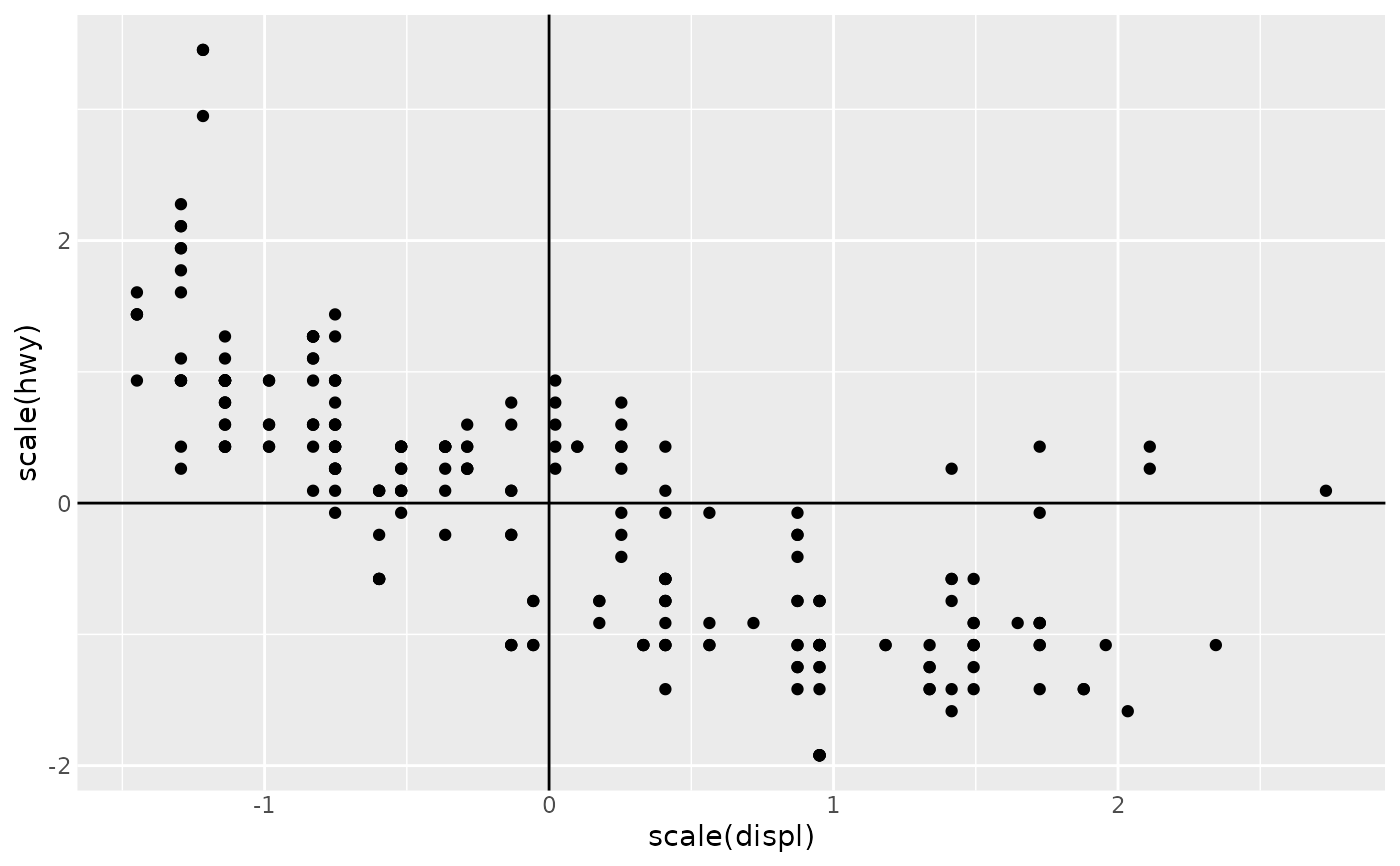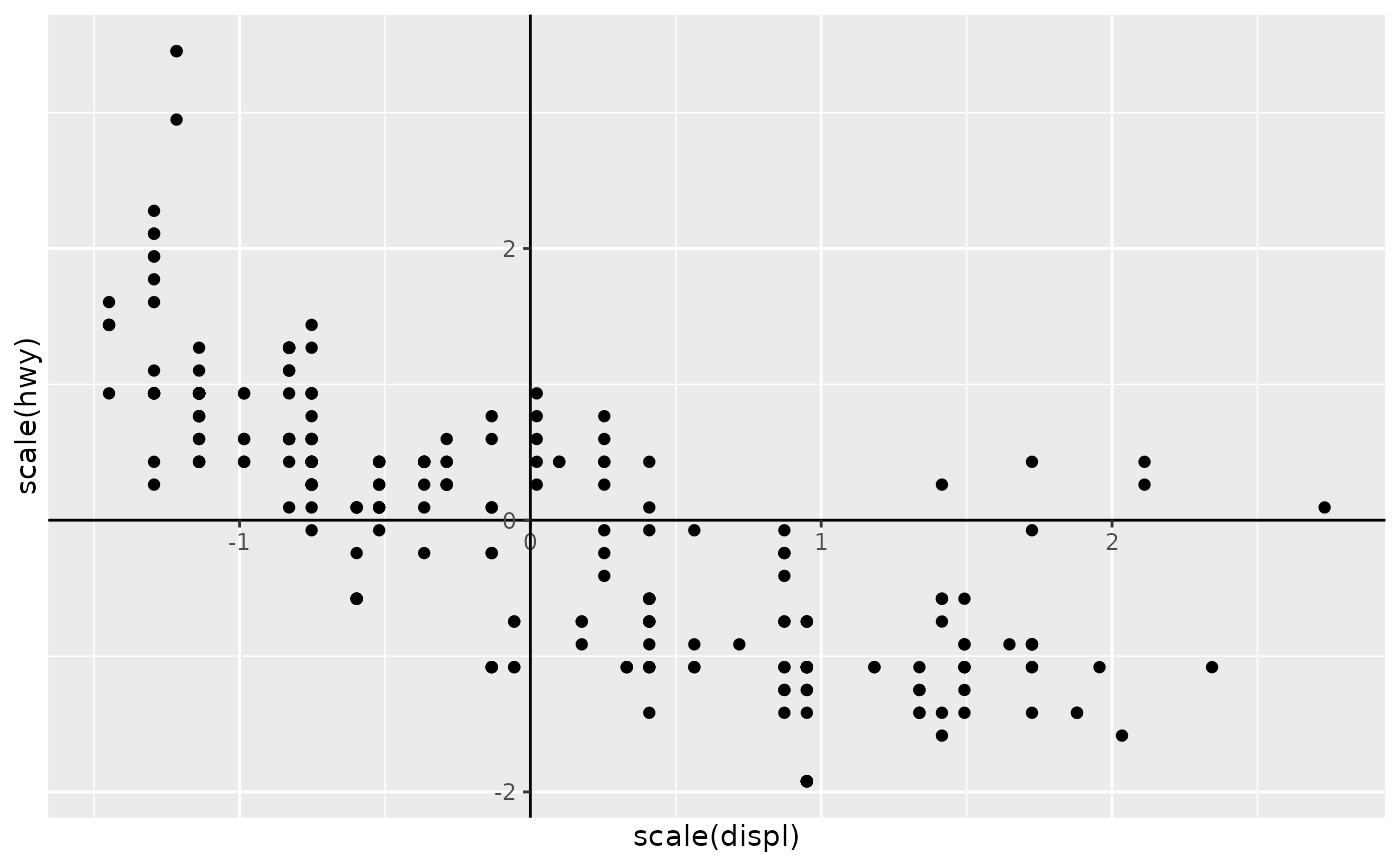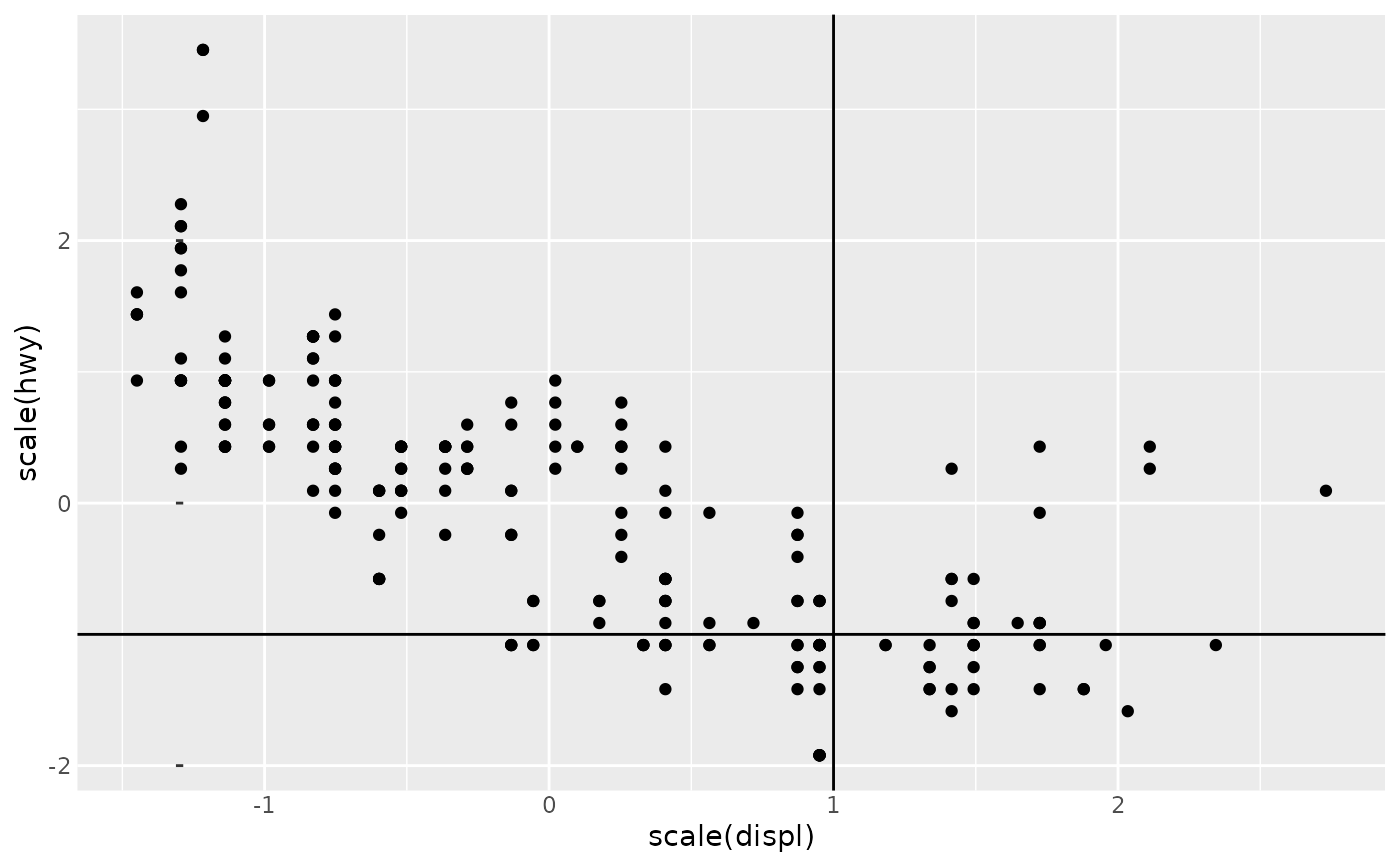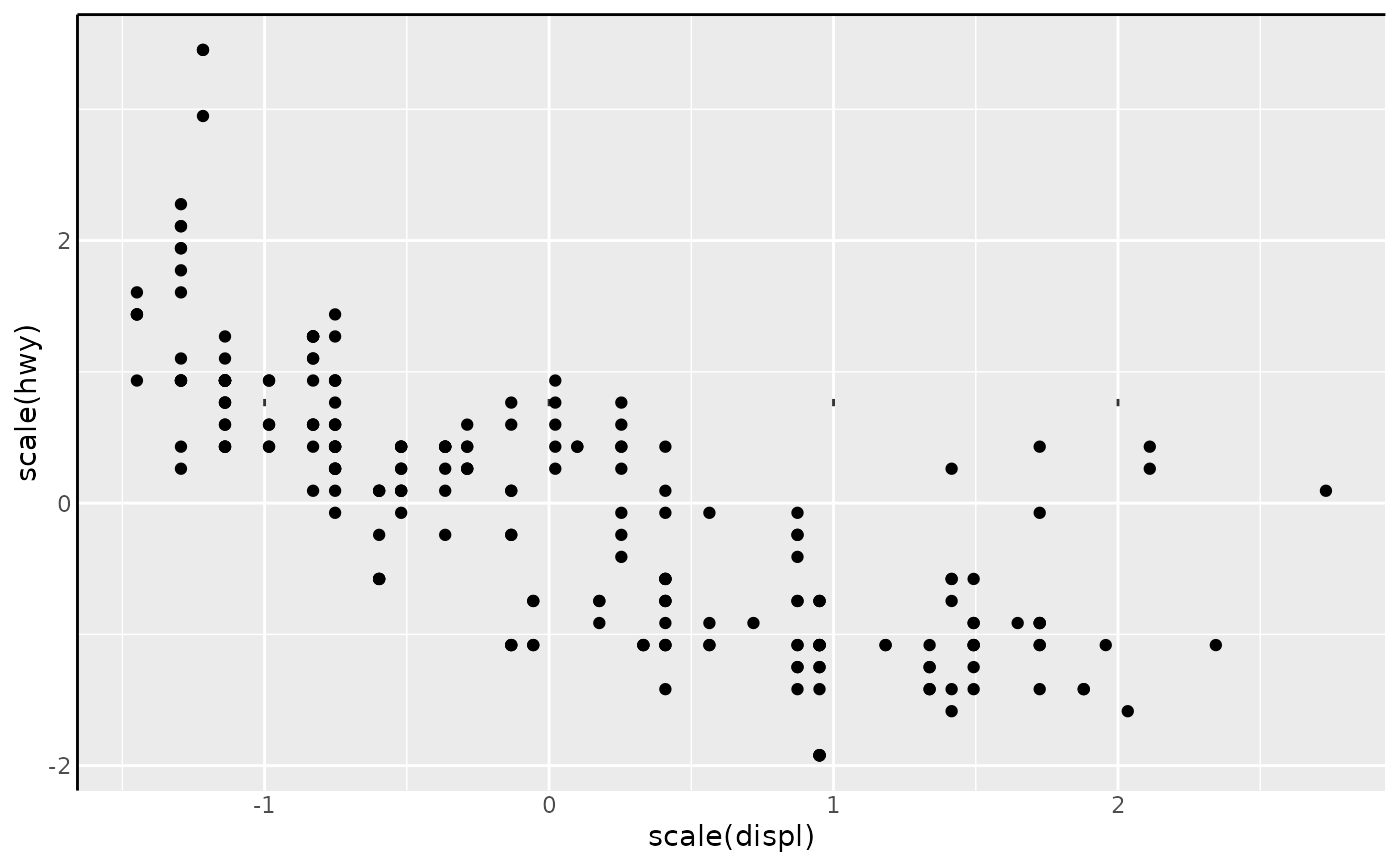This coordinate system places the plot axes at interior positions. Other than this, it behaves like coord_cartesian() or coord_fixed() (the latter if the ratio argument is set).

## Usage

coord_axes_inside(
xlim = NULL,
ylim = NULL,
xintercept = 0,
yintercept = 0,
labels_inside = FALSE,
ratio = NULL,
expand = TRUE,
default = FALSE,
clip = "on"
)

## Arguments

xlim, ylim

Limits for the x and y axes.

xintercept, yintercept

A numeric(1) for the positions where the orthogonal axes should be placed. If these are outside the bounds of the limits, the axes are placed to the nearest extreme.

labels_inside

A logical(1) when labels should be placed inside the panel along the axes (TRUE) or placed outside the panel (FALSE, default).

ratio

Either NULL, or a numeric(1) for a fixed aspect ratio, expressed as y / x.

expand

If TRUE, the default, adds a small expansion factor to the limits to ensure that data and axes don't overlap. If FALSE, limits are taken exactly from the data or xlim/ylim.

default

Is this the default coordinate system? If FALSE (the default), then replacing this coordinate system with another one creates a message alerting the user that the coordinate system is being replaced. If TRUE, that warning is suppressed.

clip

Should drawing be clipped to the extent of the plot panel? A setting of "on" (the default) means yes, and a setting of "off" means no. In most cases, the default of "on" should not be changed, as setting clip = "off" can cause unexpected results. It allows drawing of data points anywhere on the plot, including in the plot margins. If limits are set via xlim and ylim and some data points fall outside those limits, then those data points may show up in places such as the axes, the legend, the plot title, or the plot margins.

## Value

A CoordAxesInside object, which can be added to a plot.

## Examples

# A standard plot
p <- ggplot(mpg, aes(scale(displ), scale(hwy))) +
geom_point() +
theme(axis.line = element_line())

# By default, axis text is still placed outside the panel
p + coord_axes_inside()# However, this can simply be changed
p + coord_axes_inside(labels_inside = TRUE)# The place where the axes meet can be changed
p + coord_axes_inside(xintercept = 1, yintercept = -1)# Axes snap to the nearest limit when out-of-bounds
p + coord_axes_inside(xintercept = -5, yintercept = Inf, clip = "off")# Can be combined with other non-default axes
p + guides(x = "axis_minor") +
coord_axes_inside()